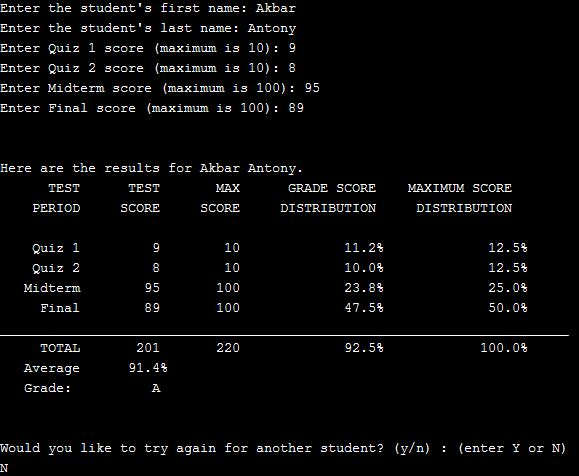SHARE
HELP

To start with, Sr2Jr’s first step is to reduce the expenses related to education. To achieve this goal Sr2Jr organized the textbook’s question and answers. Sr2Jr is community based and need your support to fill the question and answers. The question and answers posted will be available free of cost to all.Authors:
Walter Savitch ,kenrick Mock
Chapter:
Structures And Classes
Exercise:
Programming Projects
Question:1 | ISBN:9780132846813 | Edition: 5

###Question

Write a grading program for a class with the following grading policies:

a. There are two quizzes, each graded on the basis of 10 points.

b. There is one midterm exam and one final exam, each graded on the basis of

100 points.

c. The final exam counts for 50% of the grade, the midterm counts for 25%,

and the two quizzes together count for a total of 25%. (Do not forget to

normalize the quiz scores. They should be converted to a percentage before

they are averaged in.)

Any grade of 90 or more is an A, any grade of 80 or more (but less than 90) is a B, any grade of 70 or more (but less than 80) is a C, any grade of 60 or more (but less than 70) is a D, and any grade below 60 is an F. The program will read in the student’s scores and output the student’s record, which consists of two quiz and two exam scores as well as the student’s average numeric score for the entire course and final letter grade. Define and use a structure for the student record.

###Answer

``````#include <iostream>
#include <iomanip>
#include <string>
using namespace std;

{
int quiz1;
int quiz2;
int midterm;
int final;
int total;
string firstName;
string lastName;
double percent, avg;
};

void format(int n);

#define W(i) setw(17)
#define Y(i) setw(10)

int main()
{
char run_again;

do
{
getData(g);

const double Q_PERCENT = 12.5;
const double M_PERCENT = 25.0;
const double F_PERCENT = 50.0;
const int Q_SCORE = 10;
const int E_SCORE = 100;

double q1 = double(g.quiz1) / Q_SCORE * Q_PERCENT;
double q2 = double(g.quiz2) / Q_SCORE * Q_PERCENT;
double m = double(g.midterm) / E_SCORE * M_PERCENT;
double f = double(g.midterm) / E_SCORE * F_PERCENT;

g.total = g.quiz1 + g.quiz2 + g.midterm + g.final;
g.percent = q1 + q2 + m + f;

int tScore = Q_SCORE * 2 + E_SCORE * 2;
double tPercent = Q_PERCENT * 2 + M_PERCENT + F_PERCENT;

format(1);
cout << endl << endl;
cout << "Here are the results for " << g.firstName << " " << g.lastName << "." << endl;
cout << Y(i) << "TEST" << Y(i) << "TEST" << Y(i) << "MAX" << W(i) << "GRADE SCORE"
<< W(i) << "MAXIMUM SCORE" << endl;
cout << Y(i) << "PERIOD" << Y(i) << "SCORE" << Y(i) << "SCORE" << W(i) << "DISTRIBUTION"
<< W(i) << "DISTRIBUTION" << endl << endl;
cout << Y(i) << "Quiz 1" << Y(i) << g.quiz1 << Y(i) << Q_SCORE << W(i) << q1 << "%"
<< W(i) << Q_PERCENT << "%" << endl;
cout << Y(i) << "Quiz 2" << Y(i) << g.quiz2 << Y(i) << Q_SCORE << W(i) << q2 << "%"
<< W(i) << Q_PERCENT << "%" << endl;
cout << Y(i) << "Midterm" << Y(i) << g.midterm << Y(i) << E_SCORE << W(i) << m << "%"
<< W(i) << M_PERCENT << "%" << endl;
cout << Y(i) << "Final" << Y(i) << g.final << Y(i) << E_SCORE << W(i) << f << "%"
<< W(i) << F_PERCENT << "%" << endl;
cout << Y(i) << "_______________________________________________________________________\n";
cout << Y(i) << "TOTAL" << Y(i) << g.total << Y(i) << tScore << W(i) << g.percent << "%"
<< W(i) << tPercent << "%" << endl;

cout << Y(i) << "Average" << Y(i) << (double(g.total) / tScore * tPercent) << "%" << endl;
cout << Y(i) << "Grade: " << Y(i) << g.letterGrade << endl;

cout << endl << endl;
cout << "Would you like to try again for another student? (y/n) : ";
cout << "(enter Y or N)";
cout << endl;
cin >> run_again;
} while ((run_again == 'y') || (run_again == 'Y'));
system("pause");
return 0;

}

{
cout << "Enter the student's first name: ";
cin >> g.firstName;
cout << "Enter the student's last name: ";
cin >> g.lastName;
cout << "Enter Quiz 1 score (maximum is 10): ";
cin >> g.quiz1;
cout << "Enter Quiz 2 score (maximum is 10): ";
cin >> g.quiz2;
cout << "Enter Midterm score (maximum is 100): ";
cin >> g.midterm;
cout << "Enter Final score (maximum is 100): ";
cin >> g.final;

g.quiz1 = g.quiz1 > 10 ? 10 : g.quiz1;
g.quiz1 = g.quiz1 < 0 ? 0 : g.quiz1;
g.quiz2 = g.quiz2 > 10 ? 10 : g.quiz2;
g.quiz2 = g.quiz2 < 0 ? 0 : g.quiz2;
g.midterm = g.midterm > 100 ? 100 : g.midterm;
g.midterm = g.midterm < 0 ? 0 : g.midterm;
g.final = g.final > 100 ? 100 : g.final;
g.final = g.final < 0 ? 0 : g.final;

return;
}

{
if (g.percent >= 90.0)
else if (g.percent >= 80.0)
else if (g.percent >= 70.0)
else if (g.percent >= 60.0)
else
}

void format(int n)
{
cout.setf(ios::fixed);
cout.setf(ios::showpoint);
cout.precision(n);
} ``````

###Onlinehelper2020

After executed the above program code, then the result output:Post the discussion to improve the above solution.